# How To Prepare The Chapter On Motion For NSEJS

###### BySafeer PP###### Synopsis

The chapter on Motion is the basics of mechanics. For National Standard Examination For Junior Science questions from Motion are asked from kinematics. Careers360 helps you with important topics, questions and tips on how to prepare for NSEJS syllabus chapter Motion.###### Synopsis

The chapter on Motion is the basics of mechanics. For National Standard Examination For Junior Science questions from Motion are asked from kinematics. Careers360 helps you with important topics, questions and tips on how to prepare for NSEJS syllabus chapter Motion.

Motion is an important topic of NSEJS syllabus as it is the basics for other Physics chapters under NSEJS syllabus. The Class 9 NCERT chapter on motion has the topics of basic describing the term motion, distance, displacement, speed, velocity and acceleration, distance-time, velocity-time and acceleration-time graph, equations of uniformly accelerated motion and circular motion. To solve the problems of the chapter motion basic geometry and algebra are important. Solving linear equations and quadratic equations are important prerequisites for the chapter on Motion if you are appearing for NSEJS.

Also Read| NSEJS Physics Paper Analysis

## NSEJS Preparation Tips For The Chapter Motion

• Understand the equations of motion. All the three equations and related questions are important.

• Understanding basic geometry such as equations of straight line is useful to solve problems related to graphs

• Study all the graphs, questions and examples given in the NCERT book of Class 9

• Solve all the problems from NCERT exemplar for the chapter motion

• For more problems refer to Class 11 NCERT chapter Motion Along A Straight Line.

• Solve all the questions of NSEJS previous year question paper

• Solve NSEJS mock test by different online coaching institutes.

## Important Formulas

• Average speed=total distance travelled/time taken

• Average acceleration=total velocity/time taken

• Equations of uniformly accelerated motion are-

v=u+at

s=ut+at2/2

v2=u2+2as

• Distance travelled by the body in nth second

sn=u+a(2n-1)/2

• Equation of straight line

y=mx+c where m is the slope and c is the y intercept.

## Questions From NSEJS Previous Year Papers

Q-1- Two particles P1 and P2 move towards origin O, along X and Y-axes at constant speeds u1 and u2 respectively as shown in the figure. At t = 0, the particles P1 and P2 are at distances a and b respectively from O. Then the instantaneous distance between the two particles is given by the relation: (NSEJS 2018)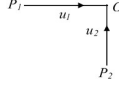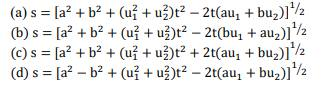Q-2- particles experience constant acceleration for 20 s after starting from rest. If it travels a distance S1 in the first 10 s and distance S2 in the next 10 s, the relation between S1 and S2 is: (NSEJS 2019)

(a) S2=3S1 (b) S1=3S2 (c) S2= 2S1 (d) S1=10S2

Q-3-The axes of a coordinate system S2are inclined at an angle θ to those of another coordinate system S1. The origins of both the systems are coinciding. A particle P1at rest in system S1, starts from point (-2, 0) and travels along the positive direction of X1 axis with uniform acceleration of 1.25 m/s2 for 4 s and stops. In system S2, particle P2, starts from rest from the origin and travels for 2 s along the positive direction of X2 axis with uniform acceleration 5 m/s2and stops. If the final distance between P1and P2 is 6 m, then the angle between +Y1 axis and +X2 axis is(NSEJS 2021)

(a) 36.8° (b) 53.2° (c) 106.8° (d) 126.8°

## NSEJS Study Materials

• NCERT Class 9 book chapter Motion

• NCERT Class 11 Chapter Motion In a Straight Line

• NCERT Exemplar problems from Motion

• NSEJS previous year question paper

The concepts of speed, distance, displacement, velocity and acceleration are prerequisite to all other physics chapters for NSEJS. So learn the chapter motion first and move to other chapters.

• Applied and interdisciplinary physics
• Mathematics
• Physics
• Physical quantities
• Classical mechanics
• Geometry
• Mechanics
• Physical phenomena
• Space
• Mechanical engineering

## Careers360 helping shape your Career for a better tomorrow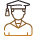#### 250M+

Students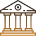#### 30,000+

Colleges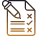#### 500+

Exams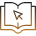E-Books